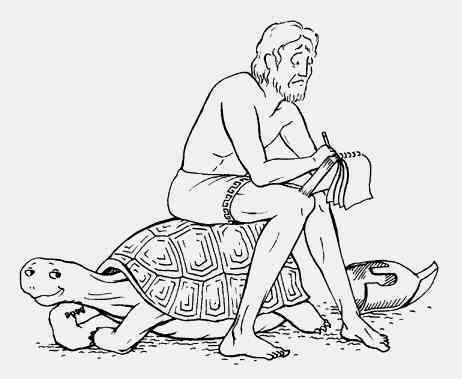# ACHILLES AND THE TORTOISE

This summer, while reading Tolstoy’s classic “War and Peace“ I came across an interesting ancient Greek puzzle. This paradox was originally devised by Zeno of Elea (ancient Greece). So, let’s see what captivated Zeno’s and Tolstoy’s mind all those years ago.

The puzzle goes like this:

Achilles is hunting a tortoise. The tortoise is in front of Achilles, so they are separated by an indefinite distance (just for this example, let’s say Achilles is 100 m behind the tortoise). Achilles is moving ten times faster than the tortoise. Both Achilles and the tortoise are in motion.By the time Achilles reached the tortoise’s starting point (the 100 m that separated him and the tortoise), the tortoise had already travelled a tenth of the initial distance (it travelled 10 m). Achilles crossed those 10 m, but the tortoise moved by 1 m. And again, Achilles crossed that 1 m, the tortoise had already travelled 0.1 m, and so on.

If we were to follow this puzzle to the end – we would see that it has no end. The distance between Achilles and the tortoise is infinitely getting smaller, which means Achilles will never reach the tortoise. How is that possible?

This contradicts with everything we know. Common sense tells us that Achilles has to reach the tortoise at one point. So what’s the problem?

We all know that motion is possible, although it contradicts Zeno’s philosophy. This is what Tolstoy said in his book:

“The absurd answer (that Achilles could never overtake the tortoise) resulted from this: that motion was arbitrarily divided into discontinuous elements, whereas the motion both of Achilles and of the tortoise was continuous.“(Leo Tolstoy)

The solution is fairly simple. For example, let’s say I want to cross a room. I know it would take me exactly 6 seconds to cross one half of the room (and since two halves make a whole, it would obviously take me 12 seconds to cross the entire room). Following Zeno’s logic, after crossing that one half, I would have to cross one half of that half (which would take me 3 seconds). After that, I would have to cross half of a half of a half (one eighth in total) which would take me 1.5 seconds, and so on I go into infinity. BUT, I know that it would take me exactly 12 seconds to cross the room. So let’s put this into an equation:

By adding infinite small pieces we get a finite sum. Therefore, I will definitely reach the other side of the room and Achilles would have definitely caught the tortoise.

Napisala: Mateja Napravnik